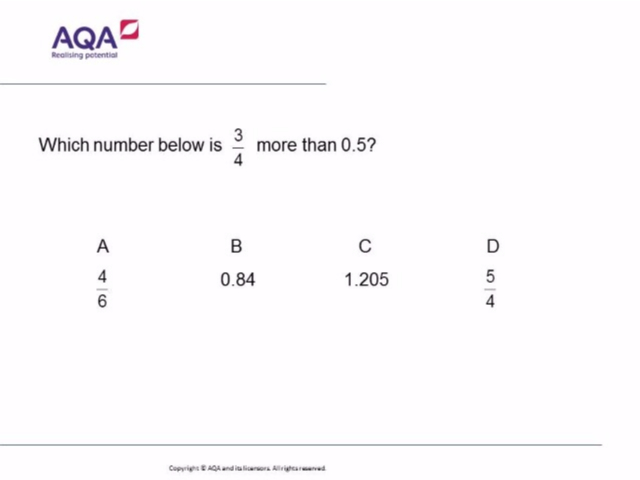# Fractions and Decimals – The Answers Revealed!

Last week’s question on fractions and decimals has been written by AQA as a taster to the kind of questions students can expect on the 2017 Maths GCSE. As some of you may know, AQA will have at least four multiple choice, diagnostic Questions on each of their GCSE Maths exams, both Higher and Foundation:Teachers correctly predicted that A would be the most common incorrect answer, but the reason for it is likely to be a big surprise. Moreover, look at the popularity of the other choices! This is a question that exposes a whole series of different misconceptions.

Now, you may think that students here have done the classic thing and changed 0.5 to ½, and then simply added the numerators and denominators together. That is certainly what my department and I thought when discussing this during out meeting last Monday. However, having read the students’ explanations, that misconception accounted for very few of them. By far the biggest misconception was a misunderstanding of what the question was actually asking, as these explanations will illustrate.

“4/6 simplifies to 2/3 the that as a decimal is 0.66 recurring and 3/4 as a decimal is 0.75 and 0.5 so 0.66 recurring is in the middle”

“because a is 0.6 and it is smaller than 3/4 which is 0.75 and more than 0.5″

“because 3/4 equals 0.75, 4/6 is equal to 0.6 and that is below 3/4 but more than 0.5″

Students have clearly read the question as “which number lies between 3/4 and 0.5?”. How frustrating is that!!!!

The other incorrect answers revealed mistakes with place value, and adding fractions, as this selection will illustrate:

B:0.34 + 0.5 = 0.84″

C:because 3/4 is 0.75 and 1.205 is above 0.5 and 0.75″